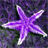# QlikView App Dev

Discussion Board for collaboration related to QlikView App Development.

Announcements
Now Live: Qlik Sense SaaS Simplified Authoring – Analytics Creation for Everyone: READ DETAILS
cancel
Showing results for
Did you mean:Creator

## calculating factors in script

Hi All,

I have a requirement is to calculate a column in Script which is as below-

Final Demand Value for Product= Demand Value * Cost * Factor -> (i have demand value and cost already present in table)

whereas my factor is another calculation

Factor (For first Year) = 1

Factor (For 2nd Year) = 1/1.09

Factor (For 3rd Year) = Factor (For 2nd Year)/1.09

Factor (For 4th Year) = Factor (For 3rd Year)/1.09

.

.

.

So on untill the Demand years for one product

How can i calculate the factor column in my script ?

Thanks

Supriya

1 Solution

Accepted SolutionsContributor III

1/pow((1+0.9),year-1)

ex:

1st year-> 1/pow((1+0.9),1-1)->1/pow((1+0.9),0)->1

2nd year-> 1/pow((1+0.9),2-1)->1/pow((1+0.9),1)->1/1.09

3rd year-> 1/pow((1+0.9),3-1)->1/pow((1+0.9),2)->1/1.09/1.09

..

.

.

.

.

.

.

.

10th year->1/pow((1+0.9),10-1)->1/pow((1+0.9),9)->1/1.09/1.09..../1.09

12 RepliesMVP

What is the formulae for Factor ??

Before develop something, think If placed (The Right information | To the right people | At the Right time | In the Right place | With the Right context)Creator
Author

Hi,

These are constants depending on demand years for one product

Factor (For first Year) = 1

Factor (For 2nd Year) = 1/1.09

Factor (For 3rd Year) = Factor (For 2nd Year)/1.09

Factor (For 4th Year) = Factor (For 3rd Year)/1.09

.

.

.

So on untill the Demand years for one productMVP

Not really sure, your expression about? You haven't indicate anything. For a while perhaps this?

Sample:

Load [Demand Value], Cost, Year From table;

Final:

"Factor Formulae" as Factor

Resident Sample Where Year = 2017;

Concatenate(Final)

"Factor Formulae"/2017 as Factor

Resident Sample Where Year = 2016;

Concatenate(Final)

"Factor Formulae"/2016 as Factor

Resident Sample Where Year = 2015;

Legend:

NoConcatenate

Load [Demand Value], Cost, Sum(Flag) as Factor_Sum Resident Final Group By [Demand Value], Cost;

Before develop something, think If placed (The Right information | To the right people | At the Right time | In the Right place | With the Right context)Creator
Author

Perhaps the concatenation wouldnt possible since my sample Data table is 25 million rows. (i am not sure though)

Also for every product i have various number for years depending on demand contract(eg - one product has been signed for next 10 years, another for 2 years, etc)

Sample:

[Demand Value],

Cost,

Year

From table;

Final table be something like

Final:

Left Keep(Sample)

Year,

If (Year= min Year, 1 , Previous('Factor')/1.09 ) as Factor

resident Sample

Group by Year;

But the factor formula doesnt seem quite working. it should be running calculation on itselfThanks

SupriyaCreator
Author

Maybe i missed one thing, My Sample Data be like

Sample:

ProductID,

[Demand Value],

Cost,

Year

From table;MVP

May be this?

Sample:

Load ProductID, [Demand Value], Cost, Year From table;

LET vMinYear = Min(Year);

Final:

Left Keep (Sample)

Year,

If (Year= \$(vMinYear), 1 , Previous('Factor')/1.09 ) as Factor

resident Sample

Group by Year;

Before develop something, think If placed (The Right information | To the right people | At the Right time | In the Right place | With the Right context)Creator
Author

Thanks Anil, but i think Previous(Factor) isnt working here since it is not getting any value. Even Same with PEEK

it was just an assumption for solution.

Also minimum year for every product would be differentMVP

In fact, Not sure how Factor is calculating? Will you attach sample data to investigate

Before develop something, think If placed (The Right information | To the right people | At the Right time | In the Right place | With the Right context)Creator
Author

Something like this -

i just need to calculate Factor column as shown in expected result